# 一、课程学习方法

## 1. 预习

1.1 打开百问网官网

​1.2 点击首页的"深入学习单片机双架构双系统项目实战线上班"

​1.3 再点击"单片机双架构双系统项目实战"，观看视频

## 3. 遇到问题

​ 3.1 浏览器打开：RTOS在线培训课程 - 最新的 - 问题 - 百问网嵌入式问答社区
​ 3.2 登陆用户名：购买课程时的手机号

​ （如果是最近报班的学员，系统可能没有录入账号，请使用报名登记的手机号注册，再联系班主任开通发帖权限）

​ 3.3 账号有问题的话，联系班主任。

# 二、指针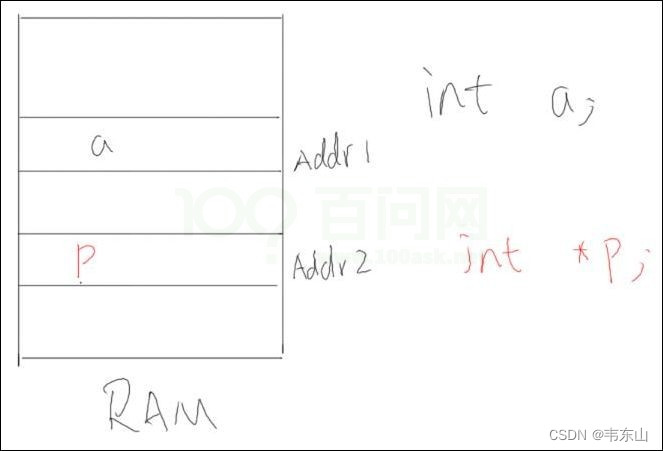P等于&a，就是P的值等于a的地址，就是图中的红字。

1. R0=123
2. R1=变量a的地址
3. 把R0的值写到R1所指示的地址去

``````int a;
int *p;
p = &a;  // p等于变量a的地址
*p = 123; // 操作变量a
``````

`unsigned int *p = 某个地址;`

` *p = 某个值;`

`unsigned int *p = 某个地址;`

``````unsigned int *p;
p = 某个地址;  // p = &a;
``````

# 三、结构体

``````struct person {
int age;
char name;
};
``````

``````wei.age = 40;
p->age = 40;
``````

• “.” : 我的
• “->”：我指向的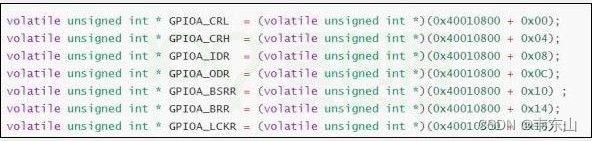“->”：指向的xxx。

gpioa->ODR = 1; 翻译就是： gpioa指向 的 ODR，等于1。

# 四、函数指针

• 保存在flash上面。

• 总得有个地址吧。

``````int a;      <===>   int add(int a, int b) {return a+b;}
int *p;     <===>   int (*pf)(int a, int b);
p = &a;    	<===>   pf = &add;
``````

int指针是变量，函数指针也是变量。

``````1. 赋值： pf = add;  // add, &add是完全一样的
2. 使用： pf(1,2 )；  //  和 (*pf) (1, 2) 是完全一样的
``````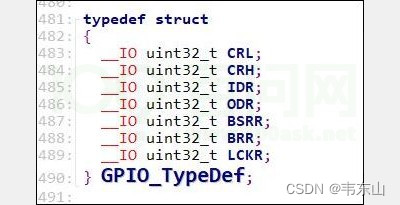# 五、链表

A要去找B， A手上得有B的地址
B要去找C， B手上得有C的地址

ABC串起来就变成了一个链。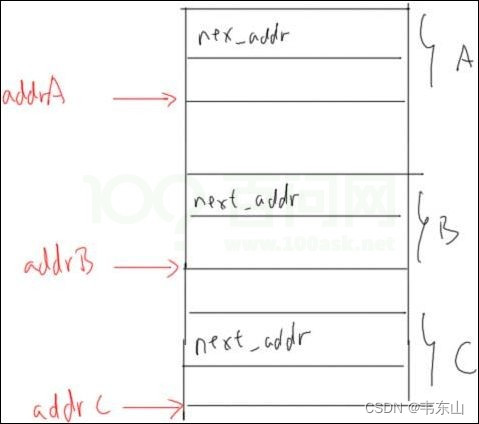A要去找B, A的手上得有B的地址，执行下面这条语句（注意箭头处）:
`A.next_addr = &B;`

B要去找C, B的手上得有C的地址，执行下条语句：
`B.next_addr = &C;`

C，他没有下线了，他手上的地址是无效的：
`C.next_addr = NULL;`

a里面有一个成员，用来保存B的地址
B里面有一个成员，用来保存C的地址

# 六、晚课学员问题

## 1. 问：

``````typedef struct
{
int a；
Char b；
Char buffer
} X_x;

X_x w;
int *p =&w;
``````

p指向结构体w的a的地址吗？

w.a,w.b,w.buffer 地址连续吗？

w占用了多少个字节？4+1+100?

``````char a;
int *p;
p = &a;
*p = 12;
``````

`*p = 12; `写4字节，但是变量a只有1字节的空间

``````char a;
int *p;
p = &a;
*p = 'A';
``````

`*p = 'A'`， 这个指令会写4个字节，错有错招，没什么后果。

``````char a;
char b;  // 多了这个变量
int *p;
p = &a;
*p = 'A';
``````

p指向结构体w的a的地址吗？ //是的

w.a,w.b,w.buffer 地址连续吗？//不连续，韦老效率，char b也被分配4字节，只用1字节，浪费3字节。

w占用了多少个字节？4+1+100? //应该是4+4+100

* : 用地址，去操作值

## 3. 问： 结构体指针gpioa的地址是在GPIO_TypeDef *gpioa;分配的。

`int a; `才分配空间，`int b`才分配空间，`int `不分配空间。

## 4. 问： 结构体定义，是保存在flash的吧？

Flash里面会保存chat这个类型吗？会保存int这个类型吗？会保存各种结构体的类型吗？

## 7. 问：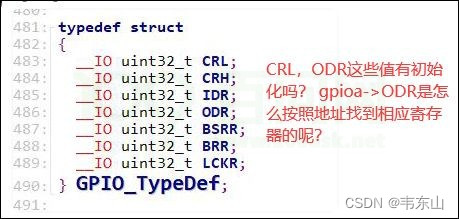## 9. 问： 老师，结构体的自引用，只能自引用指针吗？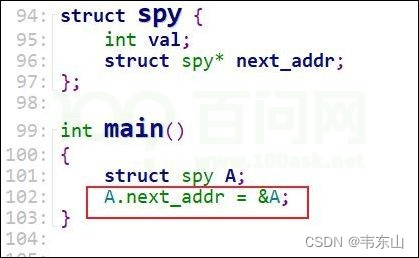``````if (A.next_addr == &A)
printf("只有一个");
``````

`struct spy* next_addr;`怎么理解？

`spy*`它是一个指针，它是结构体指针,这个结构体指针就等于某个结构体的地址。